### Home > A2C > Chapter 2 > Lesson 2.1.1 > Problem2-10

2-10.

Simplify each expression below. Be sure to show your work. (Hint: Use your understanding of the meaning of exponents to expand each expression and then simplify.) Assume that the denominators in parts (b) and (c) are not equal to zero.

1. (x3)(x2)

2.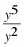3.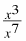4. (x2)3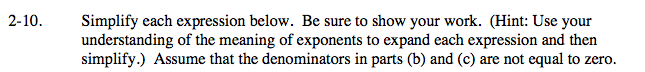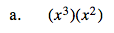Interpret the meaning of the exponents (x· x · x)(x · x), then simplify. What is the shortcut?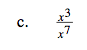What do the exponents mean?

$\text{For example: }y^{-3}=\frac{1}{y^{3}}$

Now how can you simplify the given problem?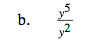What do the exponents mean? Is there a shortcut?

$\textit{\frac{y\cdot y\cdot y\cdot y\cdot y}{y\cdot y}}$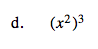Remember what each exponent means.

x6